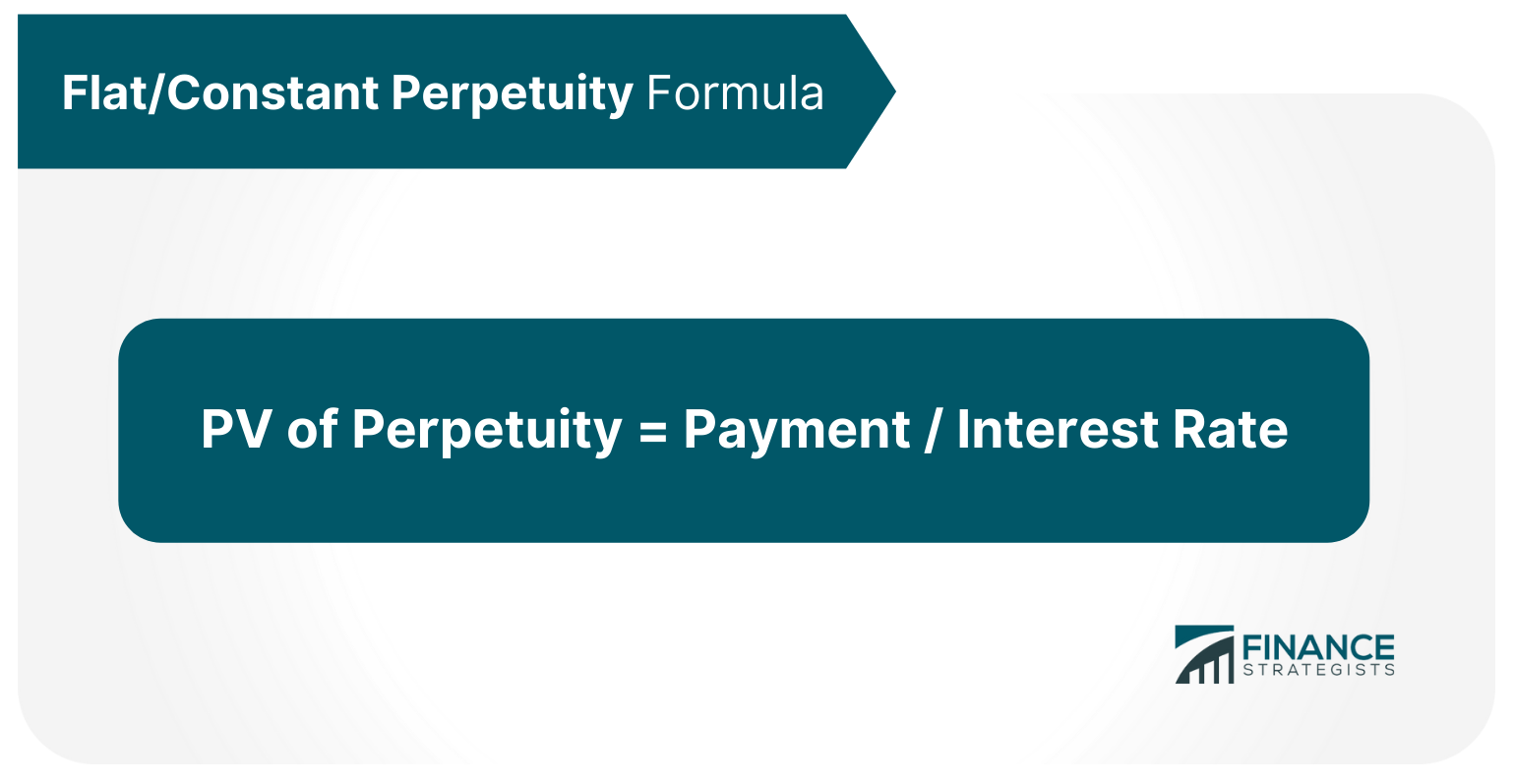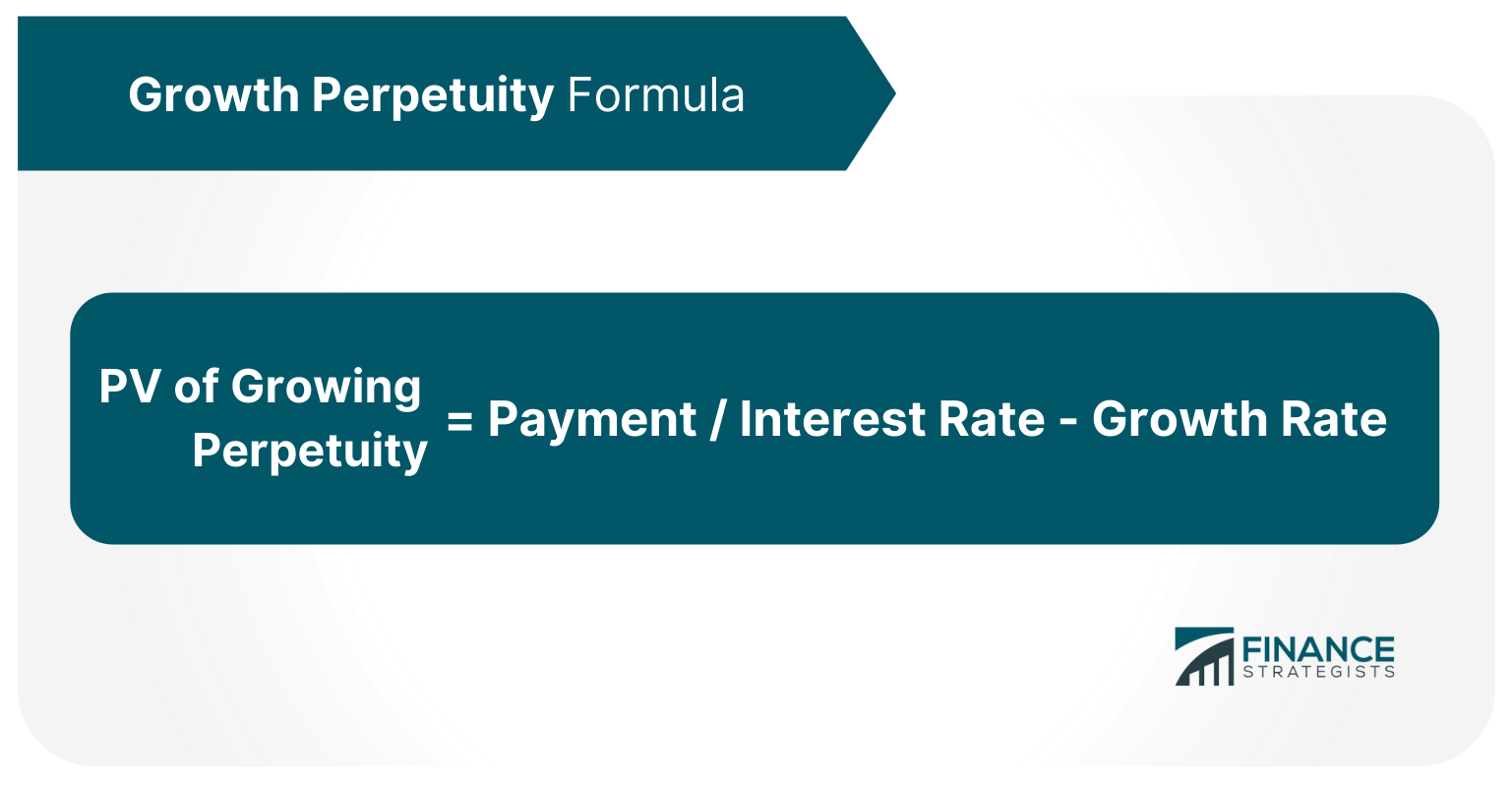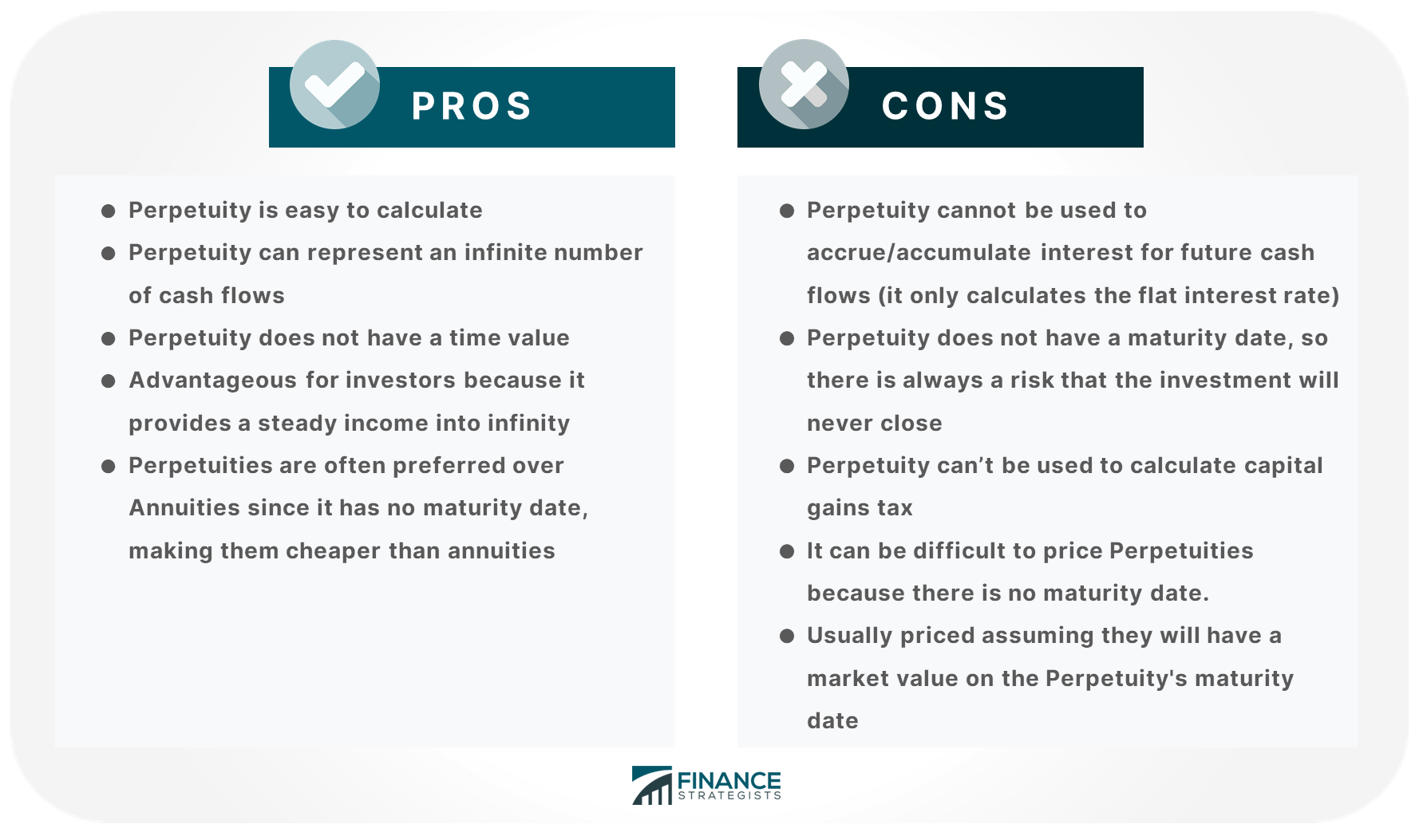# Perpetuity### Written byTrue Tamplin, BSc, CEPF® | Reviewed by Editorial Team

Updated on December 23, 2022

Perpetuity is a financial principle that calculates the value of an investment which provides a steady income into infinity.

Perpetuity can be thought of as a mirror image of an annuity since it has the same cash flows, but with no specified maturity date.

Perpetuity only specifies where the cash flow occurs, not when it happens.

## Usage of Perpetuity

Perpetuity is generally used to value assets like real estate. It is also used in infrastructure projects, where it's easy to derive future cash flows.

Perpetuity has less application in the financial services industry, but you will see it occasionally in capital budgeting decisions where a long-term project or bond issuance is being considered.

Perpetuity can also be seen as a perpetuation of the same regular and stable dividend usually associated with preferred stocks that don't have any maturity or redemption date. It works by finding the present value of an infinite series of ordinary annuity payments.

Perpetuity then discounts that figure back to today's dollars using a discount rate, which should be the appropriate risk-free rate for the period.

## Types of Perpetuity & Perpetuity Formula

There are two different annual perpetual valuations: perpetuity with flat or constant annuity and perpetuity with a growing annuity.

## Flat/Constant Perpetuity

Perpetuity with a flat or constant annuity means the same amount of money is paid each year after adjusting for inflation

## Flat/Constant Perpetuity Formula

This perpetuity formula is the simplest, and it is straightforward as it doesn’t include terminal value. It is the basic formula for the price of perpetuity.

To calculate the PV of flat perpetuity, divide the cash flows/payments by the discount rate.## Growth Perpetuity

Growth Perpetuity is a Perpetuity that grows by a certain percentage every year. The growth rate can be expressed as a simple growth rate or as a compound rate.

In Perpetuity with Growth, the cash flows are infinite and that is why it is now referred to as Perpetuity with Growth Rate instead of Perpetuity with Growing Annuity.

## Growth Perpetuity Formula

The general equation for Perpetuity with Growth Rate is:
Perpetuity with growing annuity formula is the same as Perpetuity equation except that it includes growth rate in PV formula.

This version is used to calculate the terminal value in a stream of cash flows for valuation purposes is always more complicated.## Difference of Perpetuity from Annuity

Perpetuity is the same as an annuity, but with one major difference: Perpetuity has no specified maturity date. This means that Perpetuity does not have a time value.

Because of this Perpetuity eliminates any interest calculation or compound growth calculations so it can be used to find out how much a stream of cash flows will cost at a given interest rate and growth rate.

Perpetuity can also represent an infinite stream of cash flows, but Perpetuity with Growing Annuity has a limited number of cash flows.

## Pros and Cons of Perpetuity### Pros

• Perpetuity is easy to calculate
• Perpetuity can represent an infinite number of cash flows
• Perpetuity does not have a time value
• Advantageous for investors because it provides a steady income into infinity
• Perpetuities are often preferred over Annuities since it has no maturity date, making them cheaper than annuities

### Cons

• Perpetuity cannot be used to accrue/accumulate interest for future cash flows (it only calculates the flat interest rate)
• Perpetuity does not have a maturity date, so there is always a risk that the investment will never close
• Perpetuity can’t be used to calculate capital gains tax
• It can be difficult to price Perpetuities because there is no maturity date.
• Usually priced assuming they will have a market value on the Perpetuity's maturity date

## The Bottom Line

Perpetuity is one of the most common and simple financial terms, but it can be tricky to understand because there are multiple types and formulas.

It does not accrue interest, so it cannot be used for investments that need accrued interest. It provides a steady income into infinity, but Perpetuity with Growing Annuity has a limited number of cash flows.

Perpetuities are typically preferred over Annuities because Perpetuity doesn’t have a maturity date, making them cheaper than annuities.

## Perpetuity FAQs

### What is Perpetuity?

Perpetuity refers to a stream of payments that lasts into infinity and doesn’t have a maturity date.

### How does Perpetuity work?

Perpetuities cannot accrue interest so Perpetuity calculates the discount rate based on the cash flow rate. Perpetuity does not have a maturity date, so Perpetuities do not have a time value.

### How is Perpetuity different from Annuity?

Perpetuity lasts into infinity while Annuity has a maturity date. Perpetuities do not accrue interest and Annuities do.

### What are Perpetuity's Pros and Cons?

Perpetuity does not accrue interest, has no maturity date, and has no time value. However, perpetuity cannot be used to accrue interest for future cash flows (it only calculates the flat interest rate).

### What is the Perpetuity Growth Rate?

The Perpetuity Growth Rate is the percentage by which Perpetuity Payments increase every year going into infinity.